# Dynamics Quiz 2

#### Quiz Description

In the previous quiz on dynamics, we were mainly looking at resultant forces. Here, we are going to continue by going further into the application of Newton’s third law, calculation of resultant force, etc. In a nutshell, this quiz also covers a great section of dynamics, thus, answering the two quizzes correctly is a guarantee of your success in answering dynamic questions correctly.

Dynamics is another branch of mechanics, and it is concerned with the study of forces and their relationship with motion and equilibrium. Unlike kinematics, dynamics is further divided into two types: linear and rotational dynamics. Linear dynamics studies the motion of bodies in a straight line, whereas Rotational dynamics studies the motion of bodies in a circular or curved path. We’ve heard of forces, what is a force?

A force is a push or a pull exerted on a body, and there are two categories of forces which are action-at-a-distance force, and contact force.

Dynamics is a very important topic that should be mastered to the fingertips. For this reason, this quiz, and other A level Physics quizzes are available on this platform for you to train yourself to understand better. Wish you the best as you solve the problems!

A car of mass 1500 kg travels along a horizontal road.

It accelerates steadily from 10 m / s to 25 m / s in 5.0 s.

What is the force needed to produce this acceleration?

Correct
• 300 N
• 500N
• 4500N
• 7500N

Balanced forces are acting on a moving body.

What happens to the direction of movement and to the speed of the body?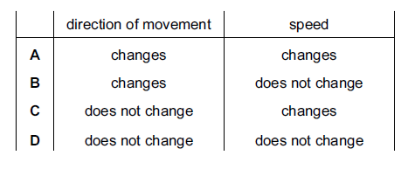Correct
• A
• B
• C
• D

A student kicks a ball horizontally along the ground. As he does so, his foot applies a force to the ball.

At the same time, the ball applies a force to his foot.

How do these forces compare?

Correct
• The force on the foot is equal to the force on the ball and in the opposite direction.
• The force on the foot is equal to the force on the ball and in the opposite direction.
• The force on the foot is smaller than the force on the ball and in the opposite direction.
• The force on the foot is smaller than the force on the ball and in the same direction.

The diagram shows an aeroplane turning in a horizontal circle at constant speed.

In which direction is there a resultant force?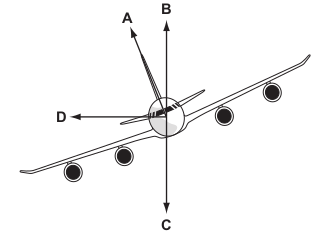Correct
• A
• B
• C
• D

A hard stone hits the ground and comes to rest almost immediately.

As the stone hits the ground, what is the direction and the size of the force acting on the ground?

Correct
• downwards and equal to the weight of the stone
• downwards and larger than the weight of the stone
• upwards and equal to the weight of the stone
• upwards and larger than the weight of the stone

A car of mass 1500 kg is towing a trailer of mass 1100 kg along a level road. The acceleration of the car is 1.30 m / s 2 .

Ignoring friction and air resistance, what is the driving force on the car?

Correct
• 1430N
• 1950N
• 2000N
• 3380N

A coin falls through the air from rest, and eventually reaches a constant speed.

Two forces P and Q act on the coin.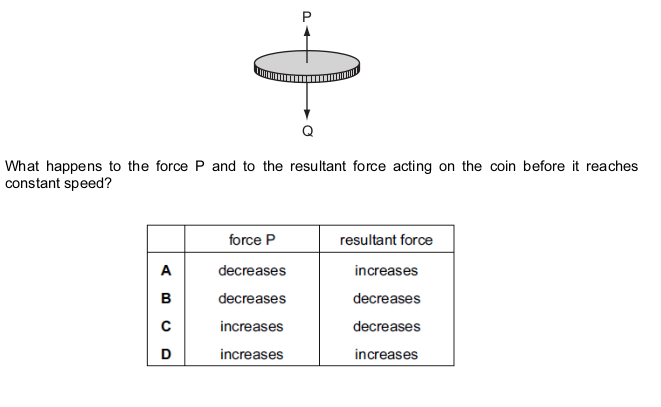Correct
• A
• B
• C
• D

The diagram shows a motorcyclist leaning over in order to move around a corner.

Which force causes him to move around the corner?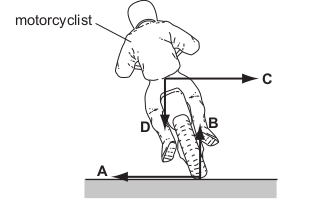Correct
• A
• B
• C
• D

An aeroplane is half way along a runway before it takes off.

The arrows show the directions of four forces acting on the aeroplane.

The drag forces on the aeroplane are negligible.

The gravitational field strength g is 10 N / kg.

The acceleration of the aeroplane is 2 m / s 2 .

What is the largest force?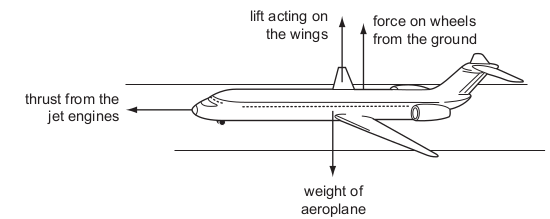Correct
• the force on the wheels from the ground
• the lift acting on the wings
• the thrust from the jet engines
• the weight of the aeroplane

The front wheel of a car is turned in a clockwise direction by the engine as the car accelerates towards the right, as shown in the diagram. There is a force of friction between the wheel and the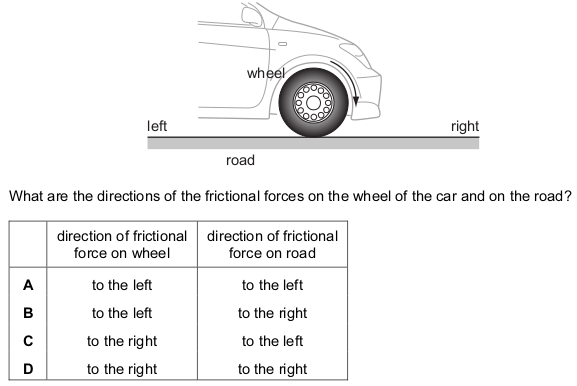Correct
• A
• B
• C
• D

The diagram shows three forces acting on a block.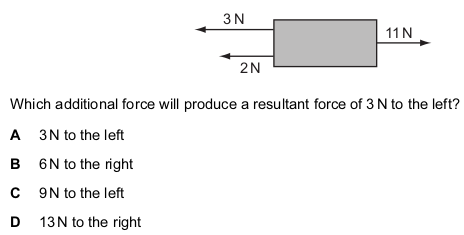Correct
• A
• B
• C
• D

The diagram shows a firework rocket.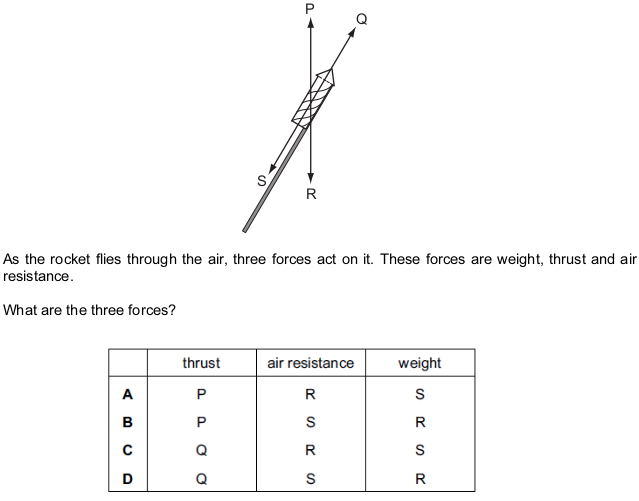Correct
• A
• B
• C
• D

An object has a mass of 15 kg. It is pushed horizontally by a force of 40 N. The frictional force is 10 N.

What is the acceleration of the object?

Correct
• 0.50 m / s 2
• 1.5 m / s 2
• 2.0 m / s 2
• 2.7 m / s 2

The propeller on a boat pushes water backwards with a force of 2000 N. The boat moves through

the water against a total resistive force of 1800 N.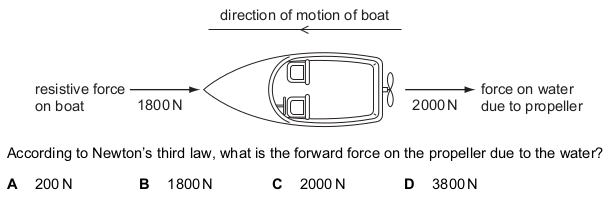Correct
• A
• B
• C
• D

An engine pulls a truck at constant speed on a level track.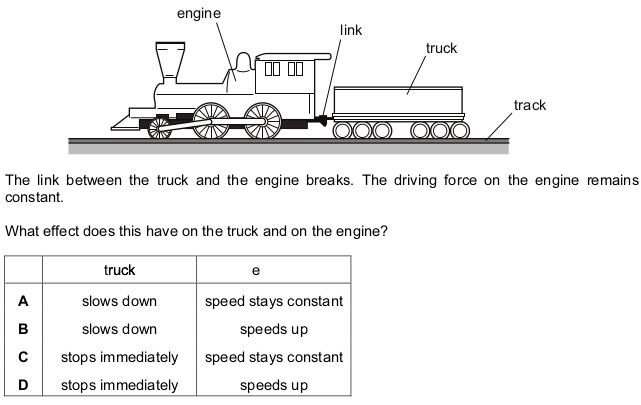Correct
• A
• B
• C
• D

A car travels along a road. The driver stops the car by pushing his foot down on the brake pedal.

What does not change if he pushes harder on the brake pedal?

Correct
• the braking distance
• the braking force
• the stopping distance
• the thinking distance

A particle P is moving in a horizontal circle about O. P moves at constant speed v.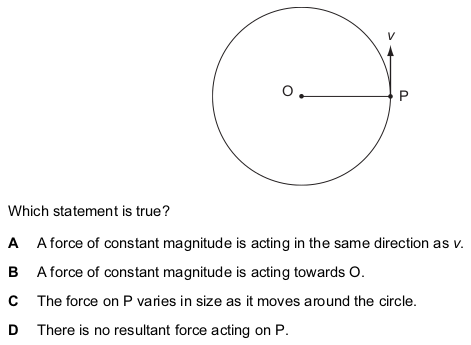Correct
• A
• B
• C
• D

A tractor pulls a trailer at a constant speed.

The tractor exerts a forward force of 1600 N on the trailer.

What is the force exerted by the trailer on the tractor?

Correct
• 0 N
• 1600 N backwards
• 1600 N forwards
• 3200 N forwards